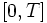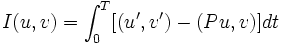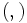# Index form

Let$\R^n$ be Euclidean space, and let$G$ be the linear space of piecewise$C^\infty$-maps from$[0,T]$ to$\R^n$. Let$H$ denote the subspace of$G$ comprising maps which are zero at the endpoints (viz maps$u$ satisfying$u(0) = u(T) = 0$).
Let$P$ be a$C^\infty$-map from$[0,T]$ into the collection of self-adjoint linear transformations on$\R^n$.
Then the index form associated with$P$ is the bilinear form on$H$ defined as follows:$I(u,v) = \int_0^T [(u',v') - (Pu,v)] dt$ where$(,)$ is the inner product on$\R^n$.